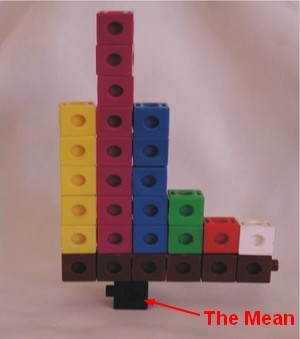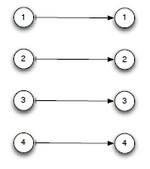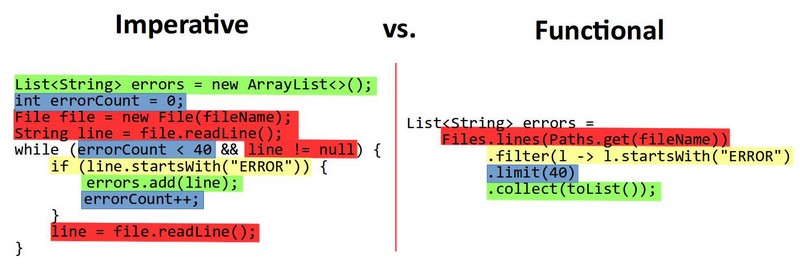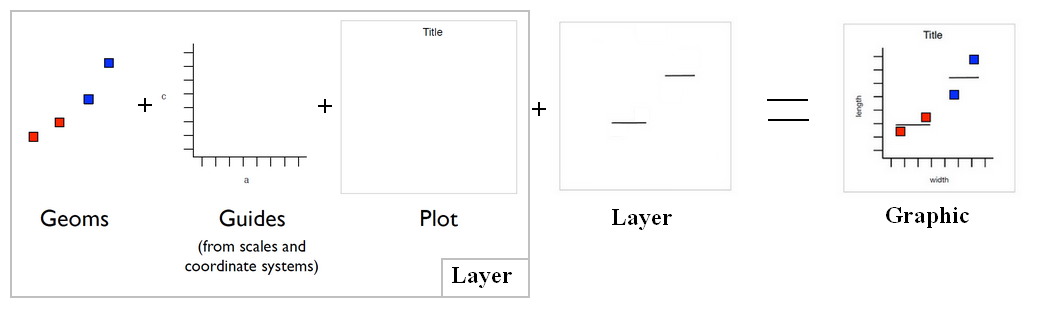# Number - Addition (or Sum, Sigma) or Total

The addition of all numbers in a set is called a total.

Sum can be use as:

It can follow the full syntax of the analytic function and in this way create Function - (Moving|Rolling|Running) Calculation

It's a binary function.

## Associative Property

An addition has an associative property and groups to the left (i.e., is left-associative). 1 + 2 + 3 is equivalent to (1 + 2 ) + 3

Example in Javascript

console.log(1 + 2 + 3); // 6
console.log((1 + 2) + 3); // 6
console.log(1 + "2" + 3); // 123
console.log((1 + "2") + 3); // 123


## Different Methods

The two arithmetic operations work on different types of operands and require different functions.

## Sigma

The symbol “sigma” is the Greek capital S and is a shortcut for “the sum of”.

• sum of x:## Analytic / Aggregate

The data come from the sample schema SH and the two next statements retrieve the same result.

### Aggregate (Group By)

select distinct CUST_LAST_NAME, sum(AMOUNT_SOLD)
from
SH.CUSTOMERS CUST,
SH.SALES SALES
where  ( CUST.CUST_ID = SALES.CUST_ID ) AND
( CUST_LAST_NAME like 'Aa%' or CUST_LAST_NAME like 'Ab%' or CUST_LAST_NAME like 'Ac%' )
group by CUST_LAST_NAME
order by CUST_LAST_NAME asc


### Analytic / Window (Over )

select distinct CUST_LAST_NAME, sum(AMOUNT_SOLD)  over (order by CUST_LAST_NAME)
from
SH.CUSTOMERS CUST,
SH.SALES SALES
where  ( CUST.CUST_ID = SALES.CUST_ID ) AND
( CUST_LAST_NAME like 'Aa%' or CUST_LAST_NAME like 'Ab%' or CUST_LAST_NAME like 'Ac%' )
order by CUST_LAST_NAME asc


### Analytic and Aggregate Statement

select
distinct CUST_LAST_NAME,
sum(AMOUNT_SOLD) ,
sum(sum(AMOUNT_SOLD) over () -- Grand Total. You must give the two times the SUM function. Otherwise you get a ORA-00979.
from
SH.CUSTOMERS CUST,
SH.SALES SALES
where  ( CUST.CUST_ID = SALES.CUST_ID ) AND
( CUST_LAST_NAME like 'Aa%' or CUST_LAST_NAME like 'Ab%' or CUST_LAST_NAME like 'Ac%' )
group by CUST_LAST_NAME
order by CUST_LAST_NAME asc


## Identity

The identity function for addition (+) is $$x+0 = x$$

## Reference

Recommended Pages(Function|Operator) - Associative Property

An operator or functionop is associative if the following holds: The importance of this to parallel evaluation can be seen if we expand this to four terms: So we can evaluate (a op b) in parallel...(Function|Operator) - Commutative property (Order doesn’t matter)

Commutative is an algebraic law. By commutative property , order in the operand doesn’t matter. Addition a + b = b + a Multiplication x . y = y . x commutativeDistribution - (Mean|Average) (M| | )

The average is a measure of center that statisticians call the mean. To calculate the mean, you add all numbers and divide the total by the number of numbers (N). The mean is not resistant. The...Function - (Aggregate | Aggregation)

Aggregate functions return a single value calculated or selected from values that are in a aggregation relationship (ie a set) This values are also known as summary because they try to summarize...Function - Binary Function/Operation

A binary operation is an scalar operation with two arguments (arity of two) that produces one value. They are creating a binary relation. the addition operator, the multiplication operator ...Function - Identity

An identity function is an algebraic law that defines when the output of the function is equal to the input. identity function. For any domain D: each domain element d map to itself: Addition...Same function name but with a different signature (list of parameters) Their will be then the same function name or an operator but that manipulates two different objects (data structure, type). ...Functional Programming - Sum

The addition (sum) function in a Functional programming context From a collection of object that return an exit status Where myMao is map where the values are integersGgplot - Weight aesthetic

The weight aesthetic represents generally a variable that must be summed.How to use the Aggregate / Window Functions (sum, avg, ) in Sqlite ?

The aggregate / window function in Sqlite. Sqlite supports the following aggregate /window function : SUM (total) AVG Max Min Rank row_number more see the Specification...